Question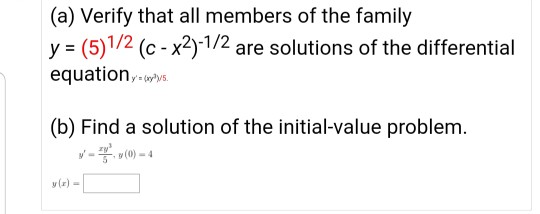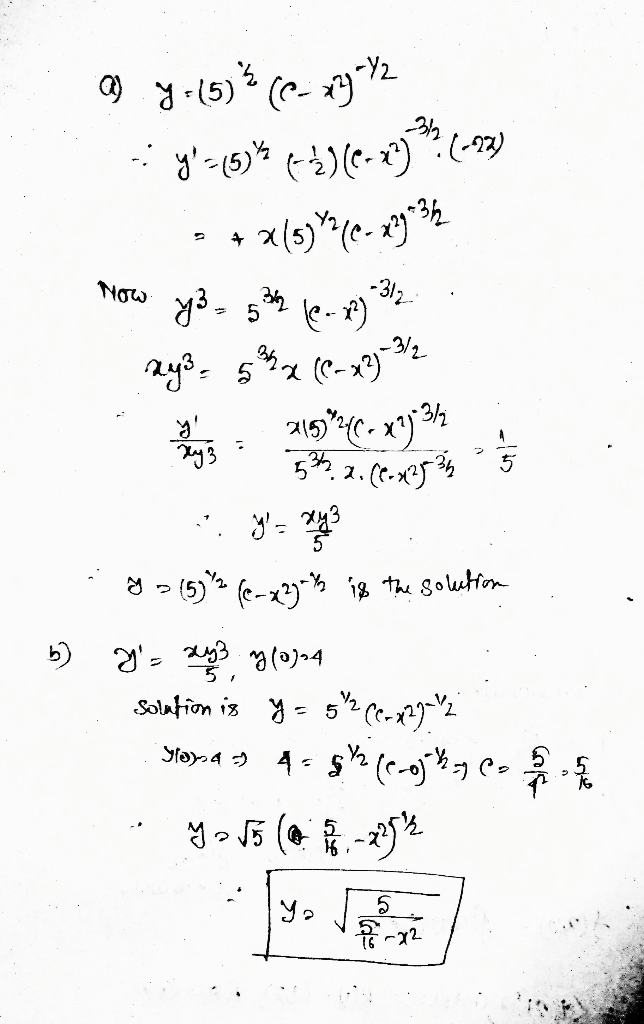#### Earn Coins

Coins can be redeemed for fabulous gifts.

Similar Homework Help Questions
• ### 7 (a) What can you about the graph of a solution of the equation y' =...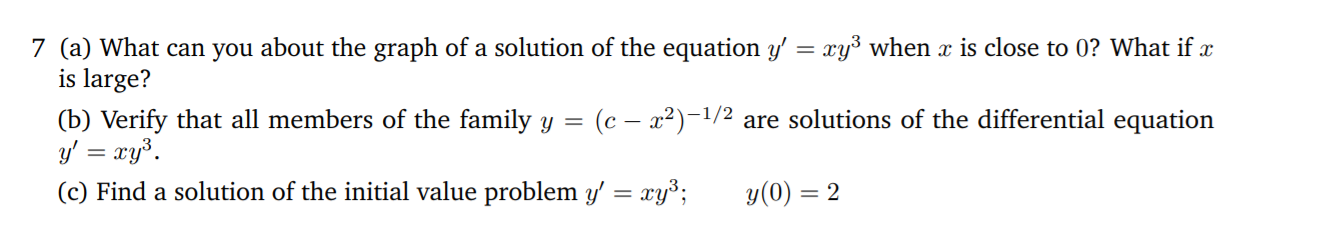7 (a) What can you about the graph of a solution of the equation y' = xy' when x is close to 0? What if x is large? (b) Verify that all members of the family y = (c - x2)-1/2 are solutions of the differential equation y' = xy. (c) Find a solution of the initial value problem y' = xyº; y(0) = 2

• ### help please! thank you! (a) Show that an members of the family y-ve-1ฐ are-lutkn-ed Medaterntial mpalii (b) Use part (a) to find a fornvula for the solution to the ini- tial value problem v (0)-2...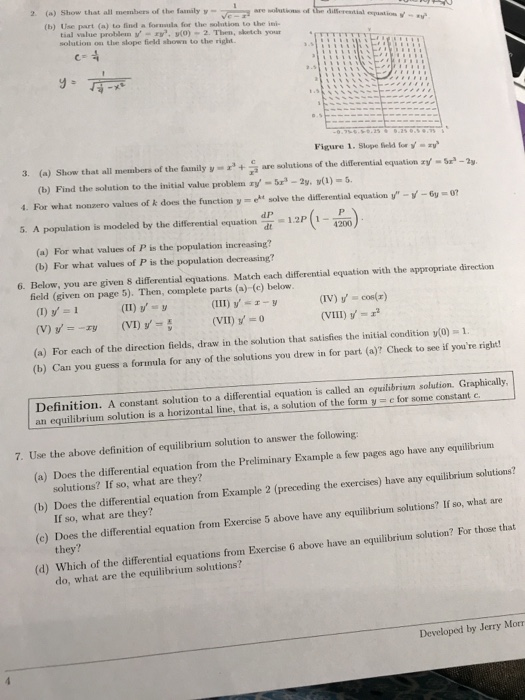help please! thank you! (a) Show that an members of the family y-ve-1ฐ are-lutkn-ed Medaterntial mpalii (b) Use part (a) to find a fornvula for the solution to the ini- tial value problem v (0)-2. Then, sketch your solution on the slope field shown to the right. 2. Figure 1. Slope field for 3. (a) Show that all members of the family yarlutions of the disferential equation (b) Find the solution to the initial value problem ry'-tra-Zy·y(1)-S. 4. For what...

• ### y = 1/(x2 + c) is a one-parameter family of solutions of the first-order DE y'...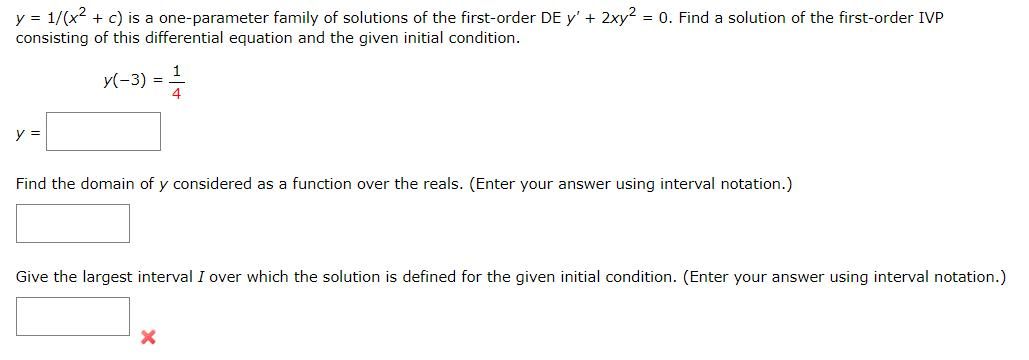y = 1/(x2 + c) is a one-parameter family of solutions of the first-order DE y' + 2xy2 = 0. Find a solution of the first-order IVP consisting of this differential equation and the given initial condition. y(-3) = y = Find the domain of y considered as a function over the reals. (Enter your answer using interval notation.) Give the largest interval I over which the solution is defined for the given initial condition. (Enter your answer using interval...

• ### 1.- The given family of solutions is the general solution of the differential equation on the...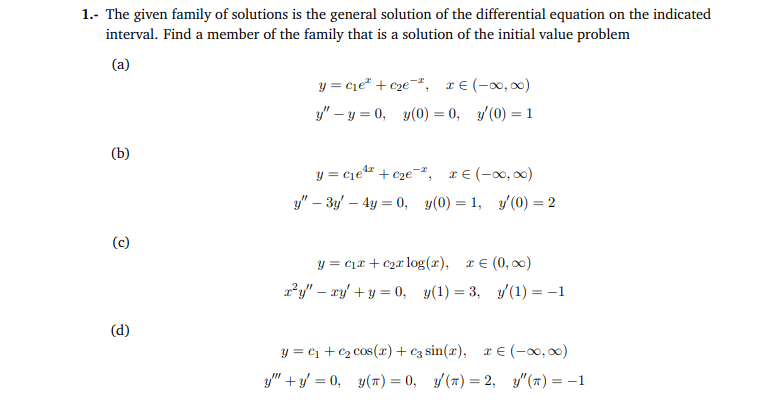1.- The given family of solutions is the general solution of the differential equation on the indicated interval. Find a member of the family that is a solution of the initial value problem (a) y = cie" + c2e-, 2€ (-0,00) y" - y = 0, y(0) = 0, 10) = 1 y=cles + cze-, 1€ (-00,00) y" – 3y – 4y = 0, y(0) = 1, y(0) = 2 Cl2 + 2x log(x), t (0, x) ry" – ry'...

• ### please answer immediately thank you 4. [-/1 Points] DETAILS SCALCCC4 7.1.006. Consider the following differential equation....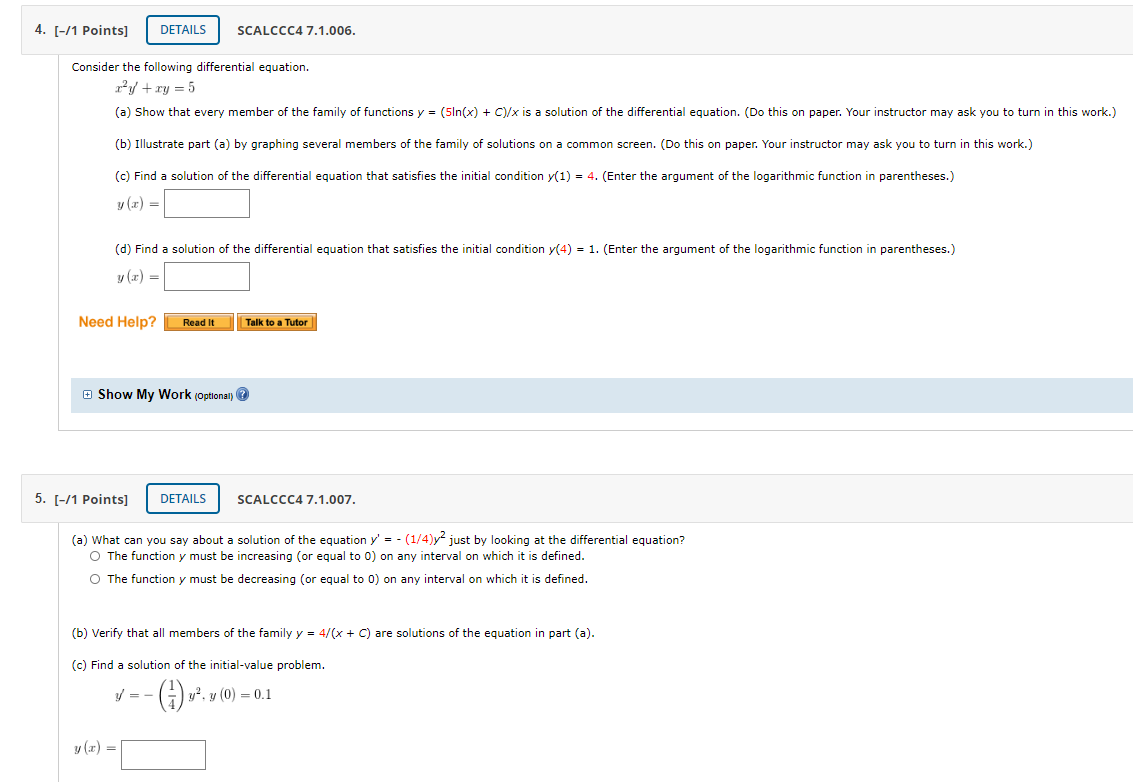please answer immediately thank you 4. [-/1 Points] DETAILS SCALCCC4 7.1.006. Consider the following differential equation. ry + y = 5 (a) Show that every member of the family of functions y = (51n(x) + C)/x is a solution of the differential equation. (Do this on paper. Your instructor may ask you to turn in this work.) (b) Illustrate part (a) by graphing several members of the family of solutions on a common screen. (Do this on paper. Your instructor...

• ### Find the solution y of the initial value problem 3"(t) = 2 (3(t). y(1) = 0,...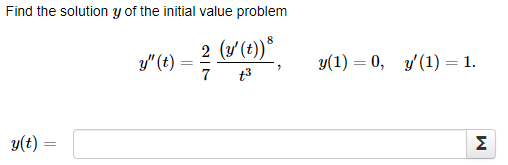Find the solution y of the initial value problem 3"(t) = 2 (3(t). y(1) = 0, y' (1) = 1. +3 g(t) = M Solve the initial value problem g(t) g” (t) + 50g (+)? = 0, y(0) = 1, y'(0) = 7. g(t) = Σ Use the reduction order method to find a second solution ya to the differential equation ty" + 12ty' +28 y = 0. knowing that the function yı(t) = + 4 is solution to that...

• ### 2. Differential equations and direction fields (a) Find the general solution to the differential equation y'...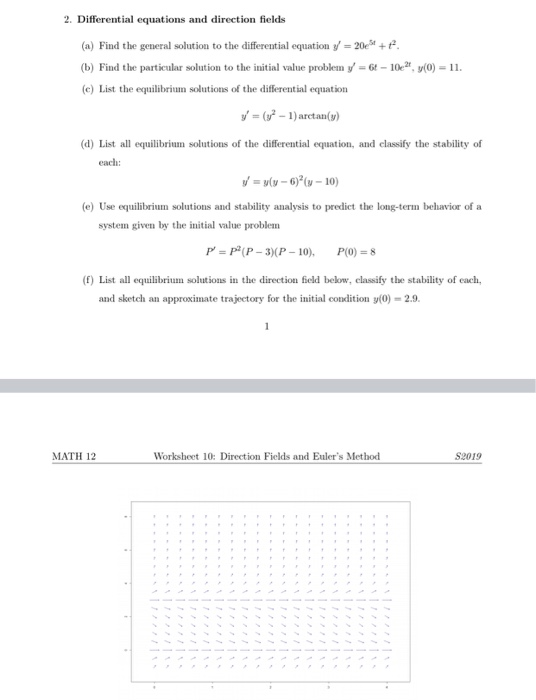2. Differential equations and direction fields (a) Find the general solution to the differential equation y' = 20e3+ + + (b) Find the particular solution to the initial value problem y' = 64 – 102, y(0) = 11. (e) List the equilibrium solutions of the differential equation V = (y2 - 1) arctan() (d) List all equilibrium solutions of the differential equation, and classify the stability of each: V = y(y - 6)(n-10) (e) Use equilibrium solutions and stability analysis...

• ### Find a differential equation whose solution is: 30. Find a 1-parameter family of solutions of the...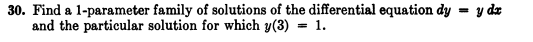Find a differential equation whose solution is: 30. Find a 1-parameter family of solutions of the differential equation dy - y dz and the particular solution for which y(3) -

• ### Consider the differential equation y' (t) = (y-4)(1 + y). a) Find the solutions that are...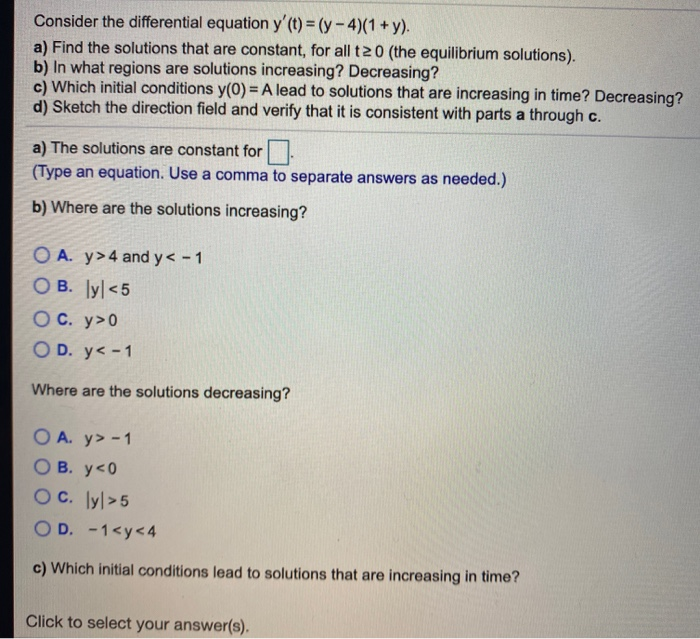Consider the differential equation y' (t) = (y-4)(1 + y). a) Find the solutions that are constant, for all t2 0 (the equilibrium solutions). b) In what regions are solutions increasing? Decreasing? c) Which initial conditions y(0) = A lead to solutions that are increasing in time? Decreasing? d) Sketch the direction field and verify that it is consistent with parts a through c. a) The solutions are constant for (Type an equation. Use a comma to separate answers as...

• ### Answer all Please! Thanks 1. Confirming Solutions to Differential Equations: Verify that each function does in fact solve the given differential equation. If there are parameters in the function (A....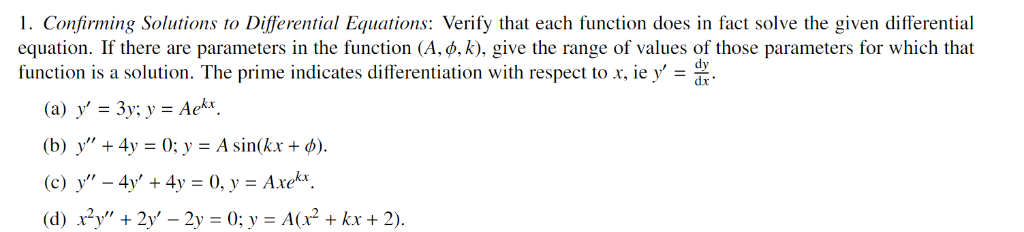Answer all Please! Thanks 1. Confirming Solutions to Differential Equations: Verify that each function does in fact solve the given differential equation. If there are parameters in the function (A. b. k), give the range of values of those parameters for which that function is a solution. The prime indicates differentiation with respect od dr' (b) y" + 4y = 0; y = A sin(kx + φ). (c) y"-4s, + 4y = 0, y = Axe . (d) x2y', +...